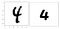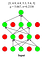# Dropout as a Bayesian Approximation: Representing Model Uncertainty in Deep Learning

Neural networks tackle a large spectrum of applications like object recognition, detection, and semantic segmentation. In image classification, a neural network predicts the object inside the image. To resolve confusing images with multiple objects, as in the next figure, the top 5 predictions are utilized.

But the top five predictions metric is different from the network confidence in its predictions. The network uncertainty is a quantitative metric revealing the network confidence in its prediction. Standard networks can easily classify the next digits as four, maybe the left image is a nine. But, they are incapable of providing a prediction uncertainty measure. For the next images, we expect higher uncertainty for the left image compared to the neat right image.Two images for digit classification. A network should classify both as ‘4’ but suffer higher uncertainty for the left image.

Dropout is a well-established procedure to regularize a neural network and limit overfitting. It is first introduced by Srivastava et al.  using a branch/prediction averaging analogy. Random neuron dropping *during training only* reduces the network generalization error.

The “dropout as a Bayesian Approximation” proposes a simple approach to quantify the neural network uncertainty. It employs dropout during *both training and testing*. The paper develops a new theoretical framework casting dropout in deep neural networks (NNs) as approximate Bayesian inference in deep Gaussian processes. The framework is developed for both classification and regression problems. This article highlights the paper finding and its applications. For simplicity purpose, regression is utilized in the following examples. Yet, classification networks are backed as well.

A regression neural network, with dropout enabled during testing, generates a different output every forward pass for the same input. In the figure below, the same input is passed six times and the network regresses to [5, 4.9, 4.8, 5.3, 5.4, 5]. The paper mathematically shows that these multiple passes are equivalent to Monte-Carlo sampling. Thus, the first and second moment (mean and variance) provides the network’s output and uncertainty respectively. In this example, the network output equals 5.067 and its uncertainty is 0.2338. High variance/standard deviation indicates high network uncertainty and vice versa. A quantitative uncertainty measure is valuable especially if further decisions are based on the network output. Human intervention is one way to address high uncertainty outputs.Neural Network with dropout enabled during testing. Multiple feed-forwards, for the same input, generate multiple outputs.

The theoretical framework employs a dropout layer before every weight layer as a Bayesian inference approximation. The dropout rate is a hyper-parameter that needs to be tuned. A small dropout rate eliminates the Monte-Carlo sampling utility. A big dropout rate can lead to divergence or at least require more iterations to converge. So, a mid-range rate like [0.1,0.2] is reasonable. Optical flow and depth estimation  are important regression problems in autonomous navigation where uncertainty estimation is valuable.

Beyond uncertainty estimation, the paper utilizes its finding in a different application. It utilizes uncertainty estimation to tune the neural network hyperparameters and reduce the generalization error. Hyper-parameters are tuned using validation splits. By employing a hyper-parameter grid search and measuring the classification accuracy or Euclidean loss metrics, the best hyperparameters get selected. In this paper, uncertainty is employed as an extra metric, besides accuracy, to tune hyper-parameters like weight-regularization coefficient. A similar followup work by Kendall et al.  used uncertainty to learn how to weight multi-task networks. A multi-term loss function, for multiple objectives/tasks, has multiple weighting hyper-parameters as in the next equation. As the number of objectives increases, tuning these weights becomes cumbersome using the naive grid search.

Loss = L1 + W2 * L2 + W3 * L3

Uncertainty quantification using dropout is the paper core contribution. A lot of applications and follow up work are based on this finding. In the medical field, Nair et al.  measure uncertainty evaluation for lesion detection and segmentation networks. In autonomous navigation, it enables semantic segmentation and depth uncertainty estimation. Gal el at. employ uncertainty estimation for active learning to boost performance from small amounts of data.

1. I find the paper contribution significant.
2. I humbling express my admiration for the theoretical foundation.
3. A round of applause is due for the released github source code.
4. From my practical experience, I have one minor negative feedback. The paper language like in “with dropout applied before every weight layer” gives the impression that dropout layers can be simply placed before every trainable layer. This confused me; probably because I am a “deep learning generation”. I prefer “ before every fully-connected layer”. A convolution layer is also a weight layer, yet it requires a different handling.
5. Based on the previous comment, I must mention followup papers that extend the current theoretical foundation to CNNs and RNNs

Dropout: a simple way to prevent neural networks from overfitting

 What Uncertainties Do We Need in Bayesian Deep Learning for Computer Vision?

 Bayesian Convolutional Neural Networks with Bernoulli Approximate Variational Inference

 A Theoretically Grounded Application of Dropout in Recurrent Neural Networks

Multi-Task Learning Using Uncertainty to Weigh Losses for Scene Geometry and Semantics

Exploring Uncertainty Measures in DeepNetworks for Multiple Sclerosis Lesion Detection and Segmentation

Deep Bayesian Active Learning with Image Data

I write reviews on computer vision papers. Writing tips are welcomed.

## More from Ahmed Taha

I write reviews on computer vision papers. Writing tips are welcomed.